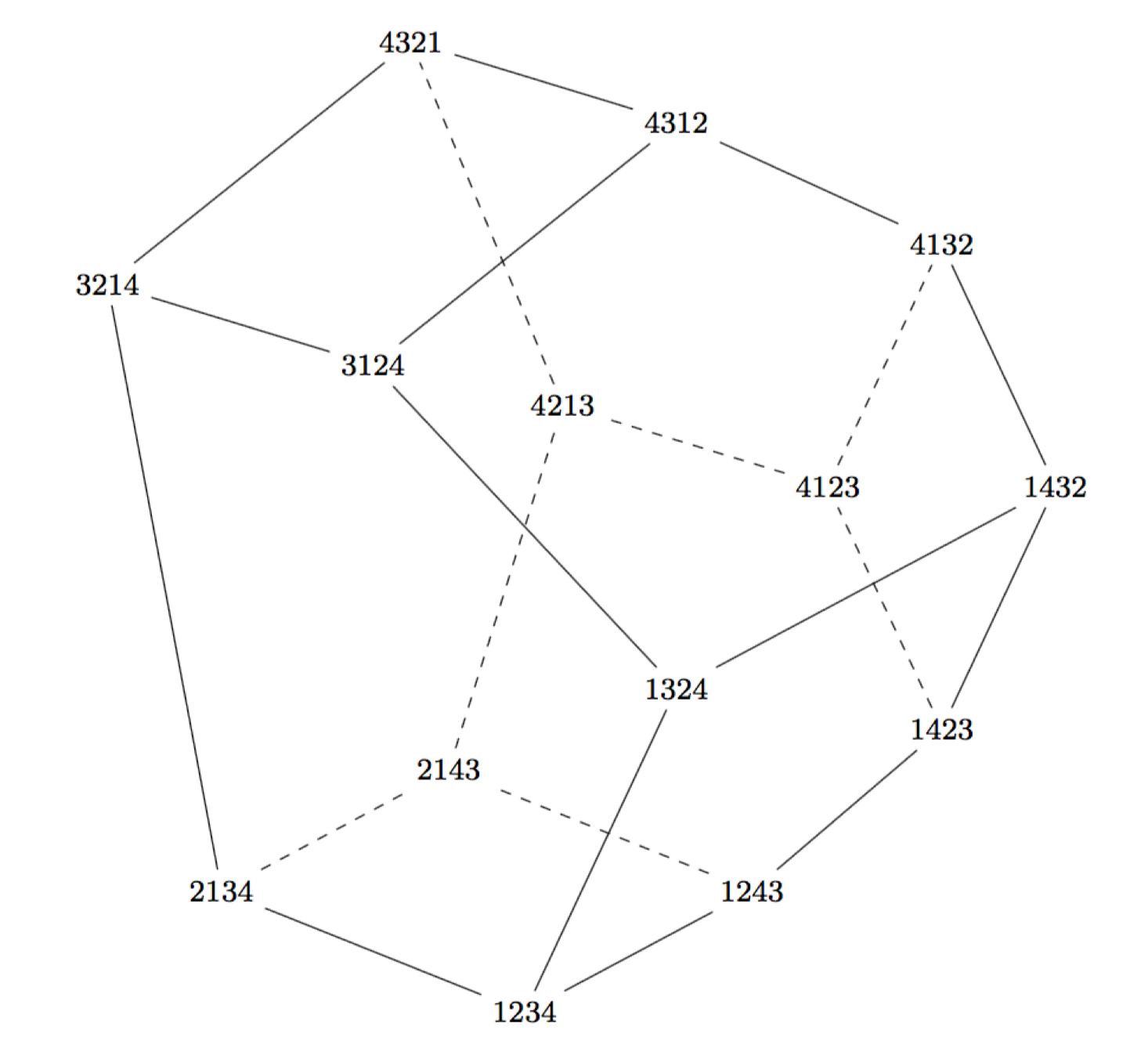Welcome

Welcome to the course web page for the Fall 2016 manifestation of MAT 526: Topics in Combinatorics at Northern Arizona University. We will be using the recently published textbook Eulerian Numbers by T. Petersen (DePaul University).

Course Info

Title: MAT 526: Topics in Combinatorics
Semester: Spring 2016
Credits: 3
Section: 1
Time: MWF at 11:30AM-12:20PM
Location: AMB 207

Instructor Info

Dana C. Ernst, PhD
AMB 176
10:15-11:15 MWF and 9-10 TTh (or by appointment)
dana.ernst@nau.edu
928.523.6852
dcernst.github.io/teaching/mat526f16

Proposed Topics

The tentative plan is to cover Chapters 1-6 and 11 of Eulerian Numbers, but we may cover more or less depending on time and interests. Here are the proposed topics:

• Eulerian numbers
• Binomial coefficients
• Generating functions
• Classical Eulerian numbers
• Eulerian polynomials
• Two important identities
• Exponential generating function
• Narayana numbers
• Catalan numbers
• Pattern-avoiding permutations
• Narayana numbers
• Dyck paths
• Planar binary trees
• Noncrossing partitions
• Partially ordered sets
• Basic definitions and terminology
• Labeled posets and P-partitions
• The shard intersection order
• The lattice of noncrossing partitions
• Absolute order and Noncrossing partitions
• Gamma-nonnegativity
• The idea of gamma-nonnegativity
• Gamma-nonnegativity for Eulerian numbers
• Gamma-nonnegativity for Narayana numbers
• Palindromicity, unimodality, and the gamma basis
• Computing the gamma vector
• Real roots and log-concavity
• Symmetric boolean decomposition
• Weak order, hyperplane arrangements, and the Tamari lattice
• Inversions
• The weak order
• The braid arrangement
• Euclidean hyperplane arrangements
• Products of faces and the weak order on chambers
• Set compositions
• The Tamari lattice
• Rooted planar trees and faces of the associahedron
• Refined enumeration
• The idea of a $q$-analogue
• Lattice paths by area
• Lattice paths by major index
• Euler-Mahonian distributions
• Descents and major index
• $q$-Catalan numbers
• $q$-Narayana numbers
• Dyck paths by area
• Coxeter groups
• The symmetric group
• Finite Coxeter groups: generators and relations
• $W$-Mahonian distribution
• $W$-Eulerian numbers
• Finite reflection groups and root systems
• The Coxeter arrangement and the Coxeter complex
• Action of $W$ and cosets of parabolic subgroups
• Counting faces in the Coxeter complex
• The $W$-Euler-Mahonian distribution
• The weak order
• The shard intersection orderThe Tamari lattice given by the weak order on $S_4(231)$. (Figure 5.12 in Eulerian Numbers by T. Petersen.)

Dana C. Ernst

Mathematics & Teaching

Northern Arizona University
Flagstaff, AZ
Website
928.523.6852
Instagram
Strava
GitHub
arXiv
ResearchGate
Mendeley
Impact Story
ORCID